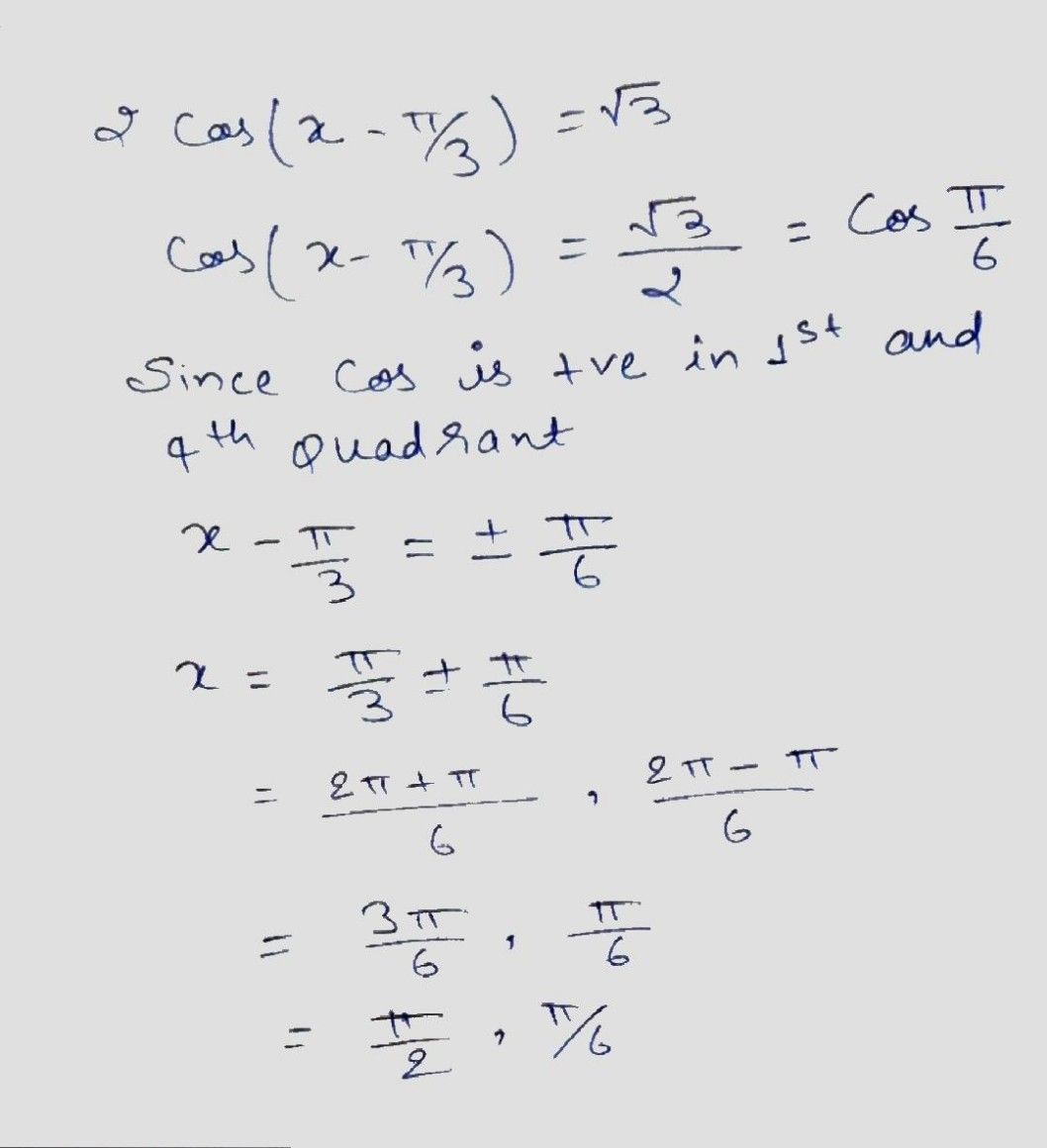Symbol
ProblemHimpunan penyelesaian persamaan $2$ $cos\left(x-\dfrac {\pi } {3}\right)$ $=\sqrt{3}$ $untuk0\leq x\leq 2\pi$ adalah
10th-13th grade
Trigonometry
Search count: 115
SolutionQanda teacher - Rashmi❣️If you have any doubt feel free to ask:) Dear, Please evaluate the answer and give gift coins(it does not reduce your coin balance.) for giving gift coins Click on evaluate. Then press the + icon 3 times(3000).
You can ask me more questions by clicking on my dp and then click on "(1:1) ask a question" and there you can ask me more questions.Student
thanks